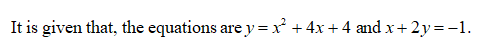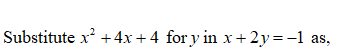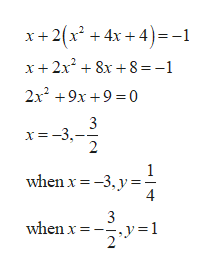# Solve. (If there is no solution, enter NO SOLUTION.)y = x2 + 4x + 4x + 2y = −1(x, y) =      (smaller x-value)(x, y) =      (larger x-value)

Question
1 views

Solve. (If there is no solution, enter NO SOLUTION.)

 y = x2 + 4x + 4 x + 2y = −1
(x, y)  =

(smaller x-value)
(x, y)  =

(larger x-value)
check_circle

Step 1Step 2...help_outlineImage Transcriptionclosex+2(x + 4x + 4)=-1 x+2x? + 8x +8 =-1 2x +9x +9 =0 3 x=-3,- when x = -3, y = 3 when x = -y=1 fullscreen

### Want to see the full answer?

See Solution

#### Want to see this answer and more?

Solutions are written by subject experts who are available 24/7. Questions are typically answered within 1 hour.*

See Solution
*Response times may vary by subject and question.
Tagged in

### Algebra